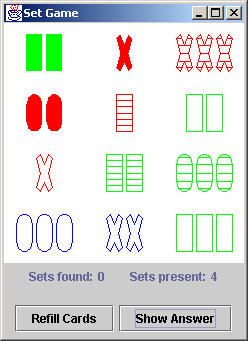# Set Puzzle

The puzzle is solved by finding sets of three cards that are all the same, or all different, across four independent "facets" or "dimensions". These dimensions are: number, shape, color, and fill. Each of these dimensions has three possible values. Number may be: one, two, or three; color may be: red, green, or blue; shape may be: rectangle, X, or O; and fill may be: open, striped, or solid.

 Example sets: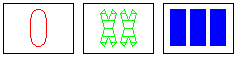a SET same: [none] different: number, color, shape, fill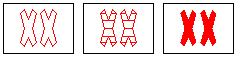a SET same: number, color, shape different: fill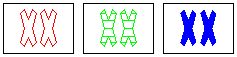a SET same: number, shape different: color, fill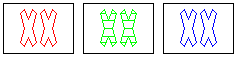NOT a set same: number, shape different: color wrong: fill To make it a set, one of the open fills needs to be solid, or the striped fill needs to be open.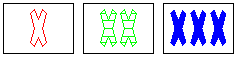a SET same: shape different: color, number, fill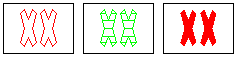NOT a set same: shape, number different: fill wrong: color To make it a set, one of the red colors needs to be blue, or the green color needs to be red.

How many cards would you expect in a Set deck?

How would you uniquely identify each card?

Can you "compute" the value of each of the four dimensions, or, does it need to be "stored"?
```public static void compute_components( int card, int[] components ) {
components = card % 3;
components = card % 9 / 3;
components = card % 27 / 9;
components = card / 27;
}
```
What kind of algorithm will give us a decision on whether 3 cards constitute a set?

```card1   0	0	1	1	2	2	0	0	1	2
card2   0	1	1	2	2	0	1	0	1	2
card3   1	1	2	2	0	0	2	0	1	2
set?	no	no	no	no	no	no	yes	yes	yes	yes
```
Above are all combinations of three 3-valued variables. Is a pattern or source of leverage evident?

Here is an implementation ...

```boolean is_a_set( int[] selected_cards ) {
int[][] cards_and_components = new int;
for (int i=0; i < 3; i++)
compute_components( selected_cards[i], cards_and_components[i] );
for (int sum, i=0; i < 4; i++) {
sum = 0;
for (int j=0; j < 3; j++)
sum += cards_and_components[j][i];
// If any of the 4 sums is not evenly divisible by 3, then the 3 cards do not form a set
if (sum % 3 != 0)
return false;
}
return true;
}
```
What kind of algorithm is necessary and sufficient to compute all the sets present in an array of 12 cards?

What is the equation for "all combinations of X things taken Y at a time"?

Here is an implementation ...

```int number_of_sets = 0;
for (int i=0;   i < cards_showing.length - 2; i++)
for (int j=i+1; j < cards_showing.length - 1; j++)
for (int k=j+1; k < cards_showing.length;     k++) {
test_set = cards_showing[i];
test_set = cards_showing[j];
test_set = cards_showing[k];
if (is_a_set( test_set )) {
number_of_sets++;